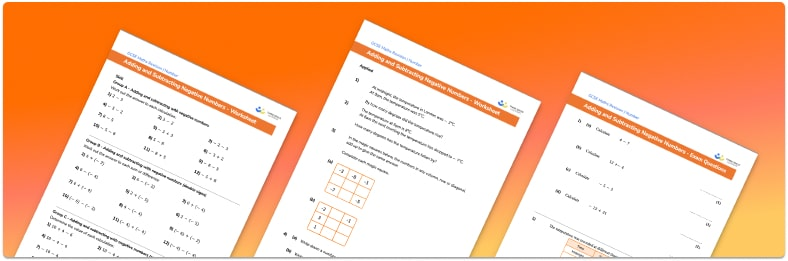# Adding and subtracting negative numbers worksheet• Section 1 of the adding and subtracting negative numbers worksheet contains 20+ skills-based adding and subtracting negative numbers  questions, in 3 groups to support differentiation
• Section 2 contains 3 applied adding and subtracting negative numbers questions with a mix of real life word problems and deeper problem solving questions
• Section 3 contains 3 foundation and higher level GCSE exam style adding and subtracting negative numbers  questions
• Answers and a mark scheme for all adding and subtracting negative numbers  questions are provided
• Questions follow variation theory with plenty of opportunities for students to work independently at their own level
• All questions and maths resources created by fully qualified expert secondary maths teachers
• Suitable for GCSE maths revision for AQA, OCR and Edexcel exam boards

• This field is for validation purposes and should be left unchanged.

You can unsubscribe at any time (each email we send will contain an easy way to unsubscribe). To find out more about how we use your data, see our privacy policy.

### Adding and subtracting negative numbers at a glance

Adding and subtracting negative numbers is an important skill and uses two of the main four operations. While whole numbers are natural numbers and include the positive integers that are not fractions, decimals or percentages, negative integers are the negative numbers that are not parts of a whole.

The best way to visualise adding and subtracting positive and negative numbers is to use a number line. When we are adding positive numbers, we move up the number line and when we are subtracting positive numbers we move down the number line. The opposite is true for negative values.

If we add a negative number, for example 3+(-2), we move down the number line whereas when we are subtracting numbers which are negative, for example 4-(-3), we move up the number line.

It is important for students to become fluent in applying the addition and subtraction facts for negative numbers.

Looking forward, students can then progress to additional Number worksheets, for example a Multiplying and Dividing Decimals worksheet or a Estimation worksheet.For more teaching and learning support on Number our GCSE maths lessons provide step by step support for all GCSE maths concepts.

## Do you have KS4 students who need more focused attention to succeed at GCSE?There will be students in your class who require individual attention to help them succeed in their maths GCSEs. In a class of 30, it’s not always easy to provide.

Help your students feel confident with exam-style questions and the strategies they’ll need to answer them correctly with our dedicated GCSE maths revision programme.

Lessons are selected to provide support where each student needs it most, and specially-trained GCSE maths tutors adapt the pitch and pace of each lesson. This ensures a personalised revision programme that raises grades and boosts confidence.

Find out more